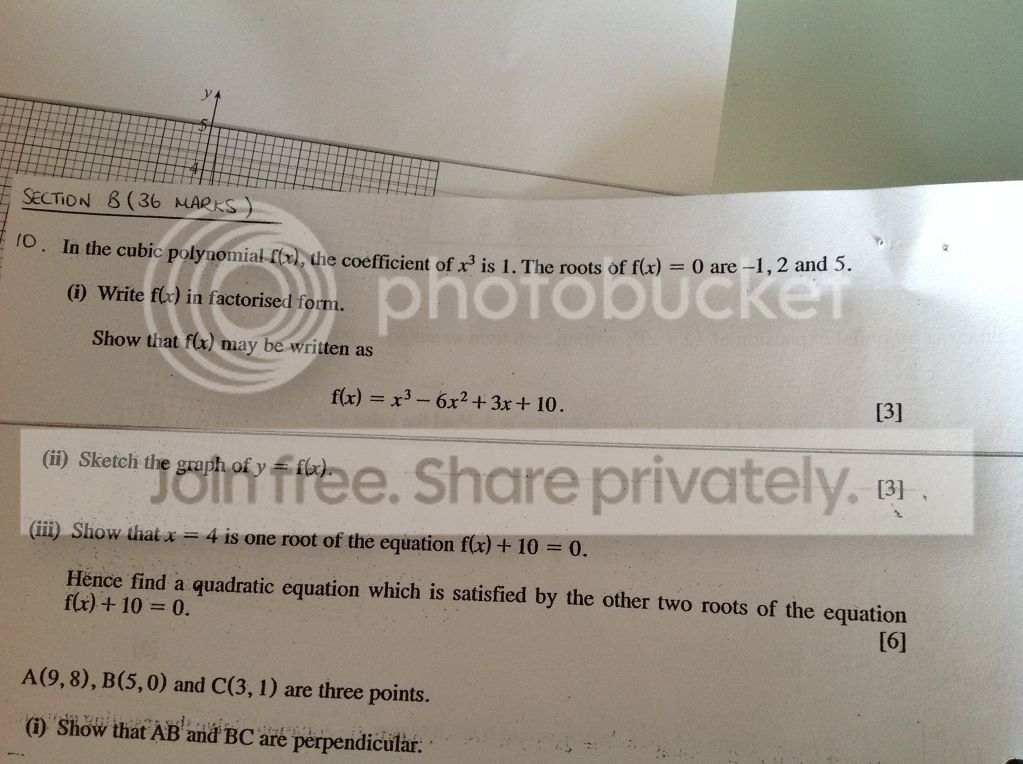Quick Maths question

Discussion in 'General Discussion' started by engstrom0304, 14 May 2012.

1. engstrom0304

Posts: 1,526

Hey guys...

So here's the question...

I was given that f(x) has an x^3 coefft of 1. The roots of f(x) are 0 , -1 , 2 and 5 ...

(i) write in factorised form and show that f(x) can be written as x^3 - 6x^2 + 3x + 10

So I factorised and got x^4 - 6x^3 + 3x^2 + 10x ... Then took out x as a factor to get the answer...

Here's the question... There were four factors... It was originally an x^4 graph... If I was going to sketch the graph then how does it work? As the fact there are 4 roots means that it wouldnt look like a cubic graph? Do I just ignore the x=0 root?

Edit: 0 doesn't give zero when substituted for x in the equation so that would mean it is not a root in the graph... But at the beginning if the question it said that 0 was a root... Now very confused :S

Last edited: 14 May 2012
2. Morbius

Posts: 2,241

If there are four roots then you would get a quartic equation, as you got in your answer, not a cubic as the question implies. There must be a mistake in the question. 0 cannot be a root of f(x).

Location: Greater London

According to (i), if it can be written as x^3 - 6x^2 + 3x + 10, then f(0)=0 is false, therefore 0 is not a root. It must be a mistake. Even Wolfram Alpha disagrees with the question: http://www.wolframalpha.com/input/?i=x^3-6x^2+3x+10

That also means the factorised form is actually (x+1)(x-2)(x-5)...

4. MuttsNutts

Posts: 679

mind melting when i have a cold so cant help today sorry

5. engstrom0304

Posts: 1,526

It does say "In the cubic polynomial f(x), the coefft of x cubed is 1 ... Does that make any difference? Is there no way that the x could be discarded as a factor to discard the x=0 root?

Last edited: 14 May 2012
6. Morbius

Posts: 2,241

You can't just arbitrarily discard factors.You always have the same number of roots as the order of the polynomial. A cubic has order 3, so you will only have 3 roots. To get the answer they're looking for, 0 cannot be a root. If 0 is a root then f(0) = 0, but according to the question, f(0) = 10.

Your best bet is to post the exact wording of the question.

7. DJMK4

Location: S.Wales

I have no idea what any of this means, so I will just sit here and smile8. marc_howarth

Location: Matlock

a cubic polynomial can never have 4 distinct roots.

9. engstrom0304

Posts: 1,526Actually having looked again I have misinterpreted the question!! It says the roots of f(x)=0 are ... Dear oh dear... I need earlier nights!

10. Josh P

Location: Cambs, UK

That means the solutions of f(x) = 0 [an equation] are -1, 2 & 5. f(x) is an expression, 'roots' implies solutions to f(x) = 0. The question doesn't mean 0 is a root.

So you know the roots, thus in factorised form, f(x) = (x-5)(x-2)(x+1). Then multiply this out: (x-5)(x-2)(x+1) = (x-5)(x^2 - x -2) = x^3 - 6x^2 + 3x +10.

11. Morbius

Posts: 2,241

Hehe, always take care reading the question.LOL! So all along it was just you misreading the question